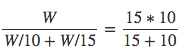# Basic Concepts of Time and WorkLast Updated on Nov 1, 2020

We have been through the concept of time and work since we are a kid. For example, while reading a book, you may have counted the time required to finish the chapter based on the rate of reading a page. Technically speaking, Work is the quantity of energy transferred from one system to another but for question-based on this topic, Work is defined as the amount of job assigned or the amount of job actually done.

## Analogy with Time-Speed-Distance

The problem with work is based on the application of the concept of the ratio of time and speed. Work is always considered as a whole or one. There exists an analogy between the time-speed-distance problems and work.

Work-based problem is more or less related to time speed and distance.

Above mentioned definition of work throws light on three important points.

Work = 1 (It is always measured as a whole) = Distance

The rate at which work is done = speed

Number of days required to do the work = Time

#### Example:

If Ram and Raman can do a job in 10 days and 15 days independently, how many days would they take to complete the same job working simultaneously?

#### Solution:

If total work is WW, Ram’s rate of working = W/10 per day and that of Raman = W/15 per day.

Thus when working simultaneously, the rate of work done = (W/10)+(W/15)*(W/10)+(W/15) and thus the time taken:
==6 Days=6 Days

#### Alternatively:

above problem can be worked out assuming work to be just 1 unit and thus eliminating the use of W.

Assume the total work to be the LCM of the days taken individually i.e. LCM of 10 & 15 i.e. 3o units of work.

Thus Ram’s rate of working = 3 units per day and Raman’s rate of working = 2 units per day.

When working simultaneously, 3+2=53+2=5 units of work is done every day and thus it would take 30/5=630/5=6 Days.

#### Note:

ALL problems or work can be solved in either way and both ways take almost the same time as there are exactly the same numbers of calculations involved. However, if you are not comfortable with fraction, the approach using LCM may seem better to you.

### Summary

In this post, we have explained the concept of time and distance in a very simple way through an example. We have also provided an alternative option for you. Apply any of the above-mentioned methods and enjoy easy solving.